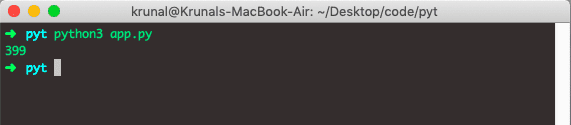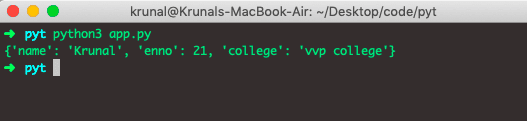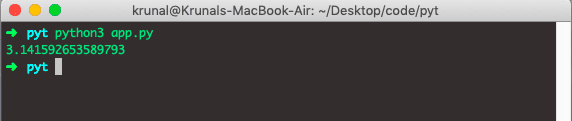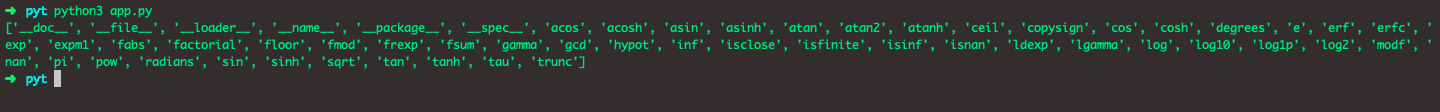# Python Modules: The Complete Guide

Python module refers to the file containing statements and definitions. Any file is a module file if that file contains the Python code and is saved as a .py extension.

## Why use Python Modules?

Python modules are files containing the set of functions you want to include in your application. We will see how to create and import that module in Python. We use the modules to break down large codebase into small, manageable, organized files.

In addition to that, modules provide the reusability of code.

We can define our most used functions in the module and import them instead of copying their definitions into different programs.

Code reusability is one of the great coding principles in any programming language.

Let’s create a module. Create a file called mod.py and add the following code.

```# mod.py

def multiply(x, y):
op = x * y
return op```

The above program returns the multiplication of two digits.

So, now mod.py is a module file. So, we can import that file into another file, use that multiply function, and get the result.

Create another file called app.py and write the following code.

```# app.py

import mod

print(mod.multiply(21, 19))```

So, we have created the app.py in the same directory as mod.py, imported the mod.py inside the app.py file, used the multiply function, passed the two parameters, and got the result.

See the output below.## How to import modules in Python?

To import modules in Python use the import keyword. You can import the definitions inside the Python module to another Python module or Python file or the interactive interpreter in Python.

If we need to import our previously defined module mod.py, we type the following code in the app.py file.

`import mod`

We can also import the direct multiply function from the mod module. See the following code.

```# app.py

from mod import multiply

print(multiply(21, 19))```

It will also give us the same output.

Python has a ton of standard modules available.

You can check out the complete list of Python standard modules.

These files are in the Lib directory inside the location where you installed Python.

Standard modules can be imported the same way we import our user-defined modules. There is no difference.

## Variables in Module

Python module can contain the functions, as already described, but variables of all types like lists, dictionaries, objects, etc.

Write the following code inside the mod.py file.

```# mod.py

student = {
'name': 'Krunal',
'enno': 21,
'college': 'vvp college'
}```

Now, import the mod.py module inside the app.py file.

```# app.py

from mod import student

print(student)```

See the output.## Naming a Module in Python

You can name the module file but must have the file extension .py.

## Import with renaming in Python

We can also give the alias to any Python module while importing that module. See the following example.

```# app.py

from mod import student as s

print(s)```

It will give you the same output as above. This is because we have renamed the module to and printed the variable from the mod.py file. So, that is how you can rename your module however you want.

## Built-in modules in Python

Many built-in modules are available like math, random, datetime, etc.

Let’s import the math module and print the value of pi.

```# app.py

from math import pi as p

print(p)```

See the output.We can also import the built-in platform module.

```# app.py

import platform
print(platform.system())```

The output is Darwin, as I am using Macbook.

## Using the dir() Function

There is a built-in function in Python to list all the function names or variable names in the module. That function name is dir(). See the following example of the dir() function in Python.

```# app.py

import math

m = dir(math)
print(m)```

The above code gives all the functions of the Math module.

See the output.That’s it for this tutorial.

This site uses Akismet to reduce spam. Learn how your comment data is processed.